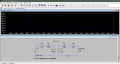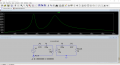# Why does mutual Coupling changes self resonant frequency and alters frequency response?

#### Lighttree18

Joined Feb 14, 2021
5
Hello all. I am conducting a simulation based report on resonant inductive coupling. I intend to calculate power transferred as a function of input frequency. For this I used the formulae for self resonant frequency and calculated a SRF of 3.283MHz using 10mH, 235pF and 0.25Ohms as my values for the RLC circuit. Using LTspiceXVII I created a schematic and the frequency analysis verified my theoretical value of 3.283MHz. However when I created another identical series RLC circuit ( with 50Ohms load) and coupled it together using the statement ( K L1 L2 1). However the frequency response showed a different frequency of 2.3215MHz this time. This resonant frequency is equal to a identical circuit but double the inductance (20mH). The mutual inductance should be equal to M = K ( sqrroot(L1*L2) ).

Why does this phenomena occur?Hence I decide to set the coupling coefficient to value of 0.5. Setting this created two peaks with current plotted on the linear X axis. According to self resonant frequency should not there be one peak?. First peak occurred at 2.68MHz at a current of 231mA and the second at 4.65Mhzat a current of 209mA.I have attached the LTSPICE file below. Your help is greatly appreciated. Thank you so much.

#### Attachments

• 1.4 KB Views: 3

#### ZCochran98

Joined Jul 24, 2018
207
The two resonances you have are the result of an effective inductance of value L - M resonating with your capacitor (Giving the 4.65 MHz peak), and an effective inductance of sum of L + M resonating with your capacitor (giving the 2.68 MHz peak). This also explains the results in the first half of your question (the difference of 0 gives zero inductance corresponding to an undefined secondary frequency, and the sum gives the resonance frequency from apparently 2L).

The way we can get this is this way: by finding the transfer function that represents this problem, I find solutions for both the real and imaginary parts. The poles for the transfer function are found by a terrible fourth-order polynomial, so I make some assumptions and approximations that let me do two things. First split the equation into the real and imaginary parts of the equation (because I am assuming $$\omega$$ to be real - not a "correct" assumption in the long run, as sufficiently-large enough values of R_L and R will make the results VERY different) to get the two parts individually. Then, as we really only care about the real part, I'll make the assumptions that $$R \ll R_L$$ and the other impedences in the circuit, so it's "close to zero," basically. Then, I define $$\Omega\equiv\omega^2$$, and solve (skipping the steps of how I got there):

$(M+L)(M-L)C^2\Omega+2LC\Omega-1=0$

for $$\Omega$$. The result is $$\Omega = \frac{1}{C(M\pm L)}$$, so $$\omega = \sqrt{\Omega} = \frac{1}{\sqrt{C(M\pm L)}}$$, which can be easily turned into its cycles-per-second equivalent.

Now, I will say this: the exact solution for that polynomial, NOT using any assumptions, gives close answers, and even gives a prediction as to the value of your decay rates (with characteristic times on the order of a few microseconds, to tens of microseconds). HOWEVER, they don't actually match! The predicted frequencies are actually 2.70 MHz and 4.59 MHz, using R = 0.25 and R_L = 50. If you were to increase R or R_L significantly, then the mismatch between my approximations using the assumptions would drastically increase.

Tl;dr: doing the math for the poles of the transfer function of that particular circuit gives some painful results that, upon taking the appropriate approximations, results in frequencies predicted as though the inductances present were L + M and L - M.

•GeBJT

#### Lighttree18

Joined Feb 14, 2021
5
The two resonances you have are the result of an effective inductance of value L - M resonating with your capacitor (Giving the 4.65 MHz peak), and an effective inductance of sum of L + M resonating with your capacitor (giving the 2.68 MHz peak). This also explains the results in the first half of your question (the difference of 0 gives zero inductance corresponding to an undefined secondary frequency, and the sum gives the resonance frequency from apparently 2L).

The way we can get this is this way: by finding the transfer function that represents this problem, I find solutions for both the real and imaginary parts. The poles for the transfer function are found by a terrible fourth-order polynomial, so I make some assumptions and approximations that let me do two things. First split the equation into the real and imaginary parts of the equation (because I am assuming $$\omega$$ to be real - not a "correct" assumption in the long run, as sufficiently-large enough values of R_L and R will make the results VERY different) to get the two parts individually. Then, as we really only care about the real part, I'll make the assumptions that $$R \ll R_L$$ and the other impedences in the circuit, so it's "close to zero," basically. Then, I define $$\Omega\equiv\omega^2$$, and solve (skipping the steps of how I got there):

$(M+L)(M-L)C^2\Omega+2LC\Omega-1=0$

for $$\Omega$$. The result is $$\Omega = \frac{1}{C(M\pm L)}$$, so $$\omega = \sqrt{\Omega} = \frac{1}{\sqrt{C(M\pm L)}}$$, which can be easily turned into its cycles-per-second equivalent.

Now, I will say this: the exact solution for that polynomial, NOT using any assumptions, gives close answers, and even gives a prediction as to the value of your decay rates (with characteristic times on the order of a few microseconds, to tens of microseconds). HOWEVER, they don't actually match! The predicted frequencies are actually 2.70 MHz and 4.59 MHz, using R = 0.25 and R_L = 50. If you were to increase R or R_L significantly, then the mismatch between my approximations using the assumptions would drastically increase.

Tl;dr: doing the math for the poles of the transfer function of that particular circuit gives some painful results that, upon taking the appropriate approximations, results in frequencies predicted as though the inductances present were L + M and L - M.

Hello ZCochran98.
I have to say I am impressed! thank you so much for a clear cut answer. This post really gives me something to start working on.
$(M+L)(M-L)C^2\Omega+2LC\Omega-1=0$
Could you kindly elaborate on this equation further?. I must need to reference this within my paper and would greatly appreciate a source or the name of the equations that derive it. Is this the Kirchoffs Voltage laws?

I am also a bit confused about the transfer function, and its poles.

Once again I can not thank you enough. You are literally a godsend.

#### ZCochran98

Joined Jul 24, 2018
207
It is indeed derived from Kirchoff's voltage laws (after converting the capacitors and inductors into impedances -> jL*omega and -j/omega*C). Specifically, this is what I did: I noted that there are 2 loops I can consider - one on the left and one on the right. By calling the current in the left loop $$I_1$$ (clockwise) and the current in the right loop $$I_2$$ (counter clockwise), I found a pair of coupled equations for the two currents. I can represent this as a matrix (to be precise, a Z-paramter matrix). I know that the transfer function that I want is V_out/Vs, and V_out = -R_L*I_2, based on how I created the current loops. So using that matrix, I inverted it (to create a Y-parameter matrix). Because part of inverting a 2x2 matrix is to divide a re-arranged matrix by the determinant of the original (a11*a22 - a12*a21), I realized that the poles would be given by the determinant of the Z-parameter matrix:

$\Delta_Z\equiv\det(Z)=z_{11}z_{22}-z_{12}z_{21}=\left(R + j\omega L- \frac{j}{\omega C}\right)\left(2R + R_L + j\omega L- \frac{j}{\omega C}\right) + \omega^2M^2 = 0$

I expanded that (a lot of arithmetic), and then made my assumptions with the resulting 4th-order polynomial (keeping in mind that any denominator of the determinant would be put into the numerator of the transfer function, contributing to the zeros, and ultimately would "cancel out" most of the fractions that would appear in the transfer function's numerator). One assumption was, basically, to divide the entire thing by R_L and replace every R/R_L with zero (because R << R_L). The other assumption was that the imaginary part of omega would be small enough that I could split the polynomial into two polynomials (even-ordered one given by things not multiplied by j and an odd-ordered on given by things that are multiplied by j). This approximation is not a mathematically-rigorous one, but "happens to" work in this case, because your resistances are low.

As it's for a paper, I would challenge you to see if you can get to the same result, based on my (hopefully not-too-vague) explanation, so I won't put all of my work down here. It's tedious, but it's doable. Start with Kirchoff's voltage laws, and keep in mind the (impedance form) of the mutual inductor (in your case) is V1 = jomega*L*I1 + jomega*M*I2, V2 = jomega*L*I2 + jomega*M*I1, find the Z-parameter matrix, then re-derivce the determinant of it, and then solve that. It's not "hard" to solve, just very tedious.

As a warning: as I mentioned, the final result I mentioned is the result of some approximations made based on the setup (low R, not-too-high R_L, etc). The actual complex value you would get for omega if you were to solve the 4th-order polynomial will be slightly different than the one you get from the approximation, but, for this case, they're in close-enough agreement.

Hope this helps, and good luck!

#### Lighttree18

Joined Feb 14, 2021
5
Wow you are truly amazing at explaining things. Its like you're reading my mind tbh. I contacted my supervisor and he told me that I am in high school and that I do not need to share the entire derivation just mentioning the source, some evaluation to reach the final approximate formulae. I know and thank you for helping me so much, and do not want to overburden you but could you please provide me with a source that's relevant to the discussion we are having? It will be greatly appreciated. THANK YOU

#### ZCochran98

Joined Jul 24, 2018
207
Glad to be of assistance!

Wikipedia's a good starting point, so you could take a look at mutual inductance and impedance there, as well as Kirchoff's laws. One thing that's helped me quite get started finding actual resources is to start at wikipedia, go to the section I'm interested in, and see what references are cited there, and, if I can, follow those references. I definitely wouldn't recommend citing Wikipedia, but it's a good place to get search terms or even references.

In regards to mutual inductances and Kirchoff's laws as they apply to them, I have a couple links that might be helpful:
http://cc.ee.ntu.edu.tw/~ultrasound/wwwroot_e/classnotes/ckt2/Chapter8.pdf